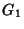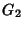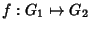## Isomorphism

Isomorphism is a very general concept which appears in several areas of mathematics. Formally, an isomorphism is Bijective Morphism. Informally, an isomorphism is a map which preserves sets and relations among elements.

A space isomorphism is a Vector Space in which addition and scalar multiplication are preserved. An isomorphism of a Topological Space is called a Homeomorphism.

Two groupsandwith binary operatorsandare isomorphic if there exists a mapwhich satisfiesAn isomorphism preserves the identities and inverses of a Group. A Group which is isomorphic to itself is called an Automorphism.